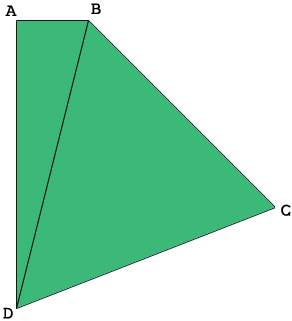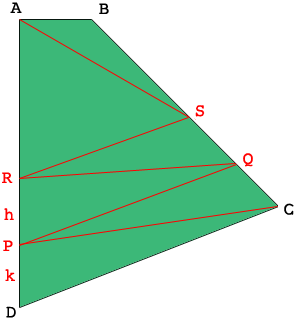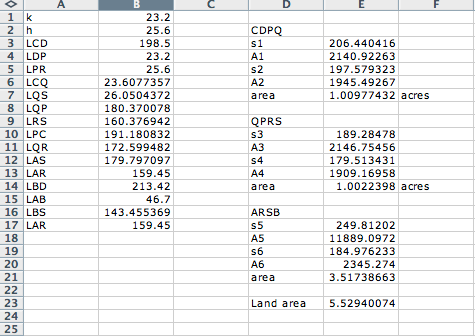SEARCH HOMEMath Central Quandaries & QueriesQuestion from Reuben: This is the measurements of my plot, A-B 46.7M, B-C 193.1, C-D 198.5 & D-A 208.25 (Clockwise naming of sides) angle A at 90 degrees. My questions is how do i subdivide this plot from the bottom having lines running parallel to C-D, eg two 2acre plots. the the remaining part becomes my compound (Upper part at line A-B)Hi Reuben,

I drew a diagram of your plot (not to scale) and added a line from $B$ to $D.$Since the angle at $A$ is a right angle I can use Pythagoras Theorem to find that the length of $BD,$ which I call $LBD$ is 213.42 meters. This way your plot is partitioned into two triangles and I know the lengths of the sides of the triangles so I can find the areas of the triangles using Heron's Formula. Heron's Formula gives the area in square meters s I divided by 4046.86 to convert it to acres. My calculations gave the area of your plot as 5.5 acres. I also used the Law of Sines and the Law of Cosines to determine the measures of the angles in these triangles. Finally I put the coordinate system on the plane with $A$ at the origin, the B on the X-axis and $D$ on the negative Y-axis. This allowed me to find the coordinated of $A, B, C,$ and $D$ and the slope of the line $CD.$

You want to subdivide your plot by lines parallel to $CD$ so I let $P$ be a point on the line $DA, k$ meters from $D,$ and $R$ be a point on $DA, h$ meters From $P.$ The lines $PQ$ and $RS$ are parallel to $CD.$The lines $PC, RQ$ and $AS$ subdivide the quadrilaterals $CDPQ, QPRS$ and $SRAB$ into triangles so I can use Heron's Formula again to calculate the areas.

The image below is a screen shot of the Microsoft Excel file I used to make the calculations.The side lengths are proceeded with an $L,$ for example the length of $CD$ is $LCD.$ The entries in columns D and E are area calculations using Heron's Formula. Hence when $k = 23.2$ meters and $h = 25.6$ meters the two plots $CDPQ$ and $PQRS$ are 1 acre each and the remaining portion, $ARSB$ is 3.5 acres.

I have attached the spreadsheet so you can modify the calculations if you wish. Download the spreadsheet. To use the spreadsheet set $h$ (entry B2) to be some small number, say $h = 10$ meters and modify $k$ until the area of $CDPQ$ is what you desire. Leaving $k$ unchanged modify $h$ until the area of $QPRS$ is what you desire.

I hope this is some use to you. Write back if my instructions are not clear.

HarleyMath Central is supported by the University of Regina and The Pacific Institute for the Mathematical Sciences.